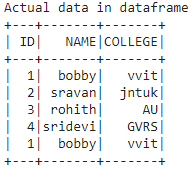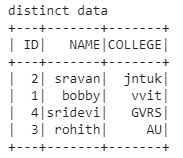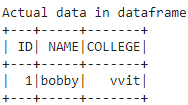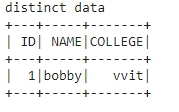Related Articles

# How to get distinct rows in dataframe using PySpark?

• Last Updated : 30 May, 2021

In this article we are going to get the distinct data from pyspark dataframe in Python, So we are going to create the dataframe using a nested list and get the distinct data.

We are going to create a dataframe from pyspark list bypassing the list to the createDataFrame() method from pyspark, then by using distinct() function we will get the distinct rows from the dataframe.

Syntax: dataframe.distinct()

Where dataframe is the dataframe name created from the nested lists using pyspark

Example 1: Python code to get the distinct data from college data in a data frame created by list of lists.

## Python3

 `# importing module``import` `pyspark`` ` `# importing sparksession from ``# pyspark.sql module``from` `pyspark.sql ``import` `SparkSession`` ` `# creating sparksession and giving``# an app name``spark ``=` `SparkSession.builder.appName(``'sparkdf'``).getOrCreate()`` ` `# list  of college data``data ``=` `[[``"1"``, ``"bobby"``, ``"vvit"``], ``        ``[``"2"``, ``"sravan"``, ``"jntuk"``],``        ``[``"3"``, ``"rohith"``, ``"AU"``],``        ``[``"4"``, ``"sridevi"``, ``"GVRS"``], ``        ``[``"1"``, ``"bobby"``, ``"vvit"``]]`` ` `# specify column names``columns ``=` `[``'ID'``, ``'NAME'``, ``'COLLEGE'``]`` ` `# creating a dataframe from the ``# lists of data``dataframe ``=` `spark.createDataFrame(data, columns)`` ` `print``(``'Actual data in dataframe'``)``dataframe.show()`

Output:Now Get the distinct rows in dataframe:

## Python3

 `print``(``'distinct data'``)`` ` `# display distinct data``dataframe.distinct().show()`

Output:Example 2: Python program to find distinct values from 1 row

## Python3

 `# importing module``import` `pyspark`` ` `# importing sparksession from ``# pyspark.sql module``from` `pyspark.sql ``import` `SparkSession`` ` `# creating sparksession and giving``# an app name``spark ``=` `SparkSession.builder.appName(``'sparkdf'``).getOrCreate()`` ` `# list  of college data``data ``=` `[[``"1"``, ``"bobby"``, ``"vvit"``]]`` ` `# specify column names``columns ``=` `[``'ID'``, ``'NAME'``, ``'COLLEGE'``]`` ` `# creating a dataframe from the ``# list of data``dataframe ``=` `spark.createDataFrame(data, columns)`` ` `print``(``'Actual data in dataframe'``)``dataframe.show()`

Output:Now Get the distinct rows in dataframe:

## Python3

 `print``(``'distinct data'``)`` ` `# display distinct data from``# the dataframe``dataframe.distinct().show()`

Output:Attention geek! Strengthen your foundations with the Python Programming Foundation Course and learn the basics.

To begin with, your interview preparations Enhance your Data Structures concepts with the Python DS Course. And to begin with your Machine Learning Journey, join the Machine Learning – Basic Level Course

My Personal Notes arrow_drop_up